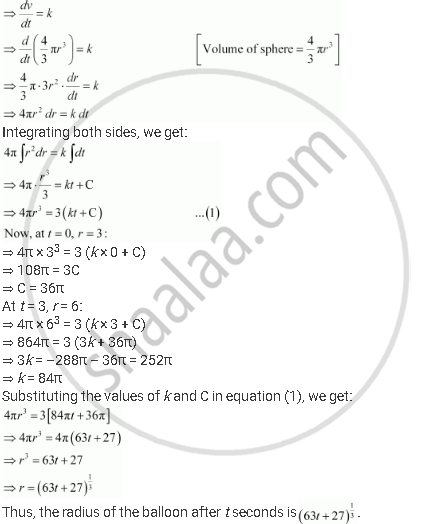Share

# The Volume of Spherical Balloon Being Inflated Changes at a Constant Rate. If Initially Its Radius is 3 Units and After 3 Seconds It is 6 Units. Find the Radius of Balloon After T Seconds. - CBSE (Commerce) Class 12 - Mathematics

ConceptMethods of Solving First Order, First Degree Differential Equations Differential Equations with Variables Separable

#### Question

The volume of spherical balloon being inflated changes at a constant rate. If initially its radius is 3 units and after 3 seconds it is 6 units. Find the radius of balloon after t seconds.

#### Solution

Let the rate of change of the volume of the balloon be k (where k is a constant).Is there an error in this question or solution?

#### APPEARS IN

NCERT Solution for Mathematics Textbook for Class 12 (2018 to Current)
Chapter 9: Differential Equations
Q: 19 | Page no. 396
Solution The Volume of Spherical Balloon Being Inflated Changes at a Constant Rate. If Initially Its Radius is 3 Units and After 3 Seconds It is 6 Units. Find the Radius of Balloon After T Seconds. Concept: Methods of Solving First Order, First Degree Differential Equations - Differential Equations with Variables Separable.
S